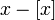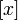# Fractional part

Jump to: navigation, search
This article is about a particular function from a subset of the real numbers to the real numbers. Information about the function, including its domain, range, and key data relating to graphing, differentiation, and integration, is presented in the article.
View a complete list of particular functions on this wiki

## Definition

The fractional part is a function from the reals to the reals that sends every real number$x$ to$x - [x]$ where$[x]$ denotes the greatest integer function of$x$.

## Key data

Item Value
default domain all real numbers
range$[0,1)$, i.e., the set$\{ x \mid 0 \le x < 1 \}$
absolute maximum value: does not exist, but the lub of the range is 1, absolute minimum value: 0
continuity The function is continuous everywhere except at integers. At any integer, the left hand limit of the function is 1, and the right hand limit is 0. In particular, the function is right continuous at all points (including integers) and not left continuous at integers.
period The function has a period of 1.
derivative the function is differentiable everywhere except at integers, and at all points other than integers, the derivative is 1.
at integers, the right hand derivative is 1, and the left hand derivative does not exist.
antiderivative Fill this in later -- a piecewise parabola